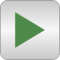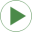# SolveOneNonlinearEquation

Demonstrate how to solve one non-linear algebraic equation in one unknown# Package ContentsInverse_sine Solve y = A*sin(w*x) for x, given yInverse_sh_T Solve h = h_T(T), s = s_T(T) for T, if h or s is given for ideal gas NASAInverseIncompressible_sh_T Inverse computation for incompressible mediaInverse_sh_TX Solve h = h_TX(TX) for T, if h is given for ideal gas NASA

# Information

This information is part of the Modelica Standard Library maintained by the Modelica Association.

This package demonstrates how to solve one non-linear algebraic equation in one unknown with function Modelica.Media.Common.OneNonLinearEquation.